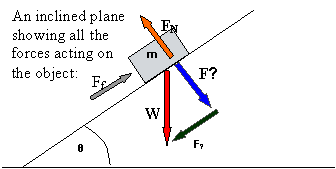HOME COURSES PREVIEW REVIEW ABOUT CONTACTTOLL-FREE INFO & ORDERING: M-F: 9am-5pm (PST): (877) RAPID-10

 Quick Search: Keywords:

 Rapid Learning Member Area:Note: If you are a legacy user of chemistry24 members, please request a new login access to the premium server with your full name and old login email via vip@rapidlearningcenter.com

 Rapid Courses Catalog : Physics in 24 Hours Chemistry in 24 Hours Biology in 24 Hours Mathematics in 24 Hours

Weekly Physics Tips:
Want to become a top gun in your class? How about study less yet score high? Sign up this Physics Survival Weekly to learn how. Designed specifically for students who are taking physics, this free newsletter will show you how to survive and excel in class! Weekly topics include:
• How to Study Physics Effectively
• How to Read Physics Textbooks Easily
• How to Solve Physics Problems Systematically
• How to Score High on Physics Exams Strategically
• How to Master Physics Rapidly
Each week, you will receive study tips on the topics above and visual tutorial or study template to enhance your physics learning. Enter your name and email below to subscribe free:

 Physics Study Lounge These study sheets are for quick review on the subjects. Refer to our rapid courses for comprehensive review.     - Basic Skills in Physics     - Basic Math for Physics     - How to Solve Physics Problems     - Newton's Laws Study Guide     - ElectroMagnetism Quick Review     - Atomic Physics At-A-Glance     - Core Concepts in Nuclear Physics     - Special Relativity Overview

 Other Related Sites
 Note: For course links to launch, disable popup blockers or hold the ctrl key while clicking the link.

Newton’s Laws Study Guide

Key Physics Terms

Vector: A quantity that represents magnitude (size) and direction.  It is usually represented with an arrow to indicate the appropriate direction.  They may or may not be drawn to scale.
Resultant: the result of adding two or more vectors; vector sum.
Vector Component: the parts into which a vector can be separated and that act in different directions from the vector.
Static Equilibrium:  A motionless state where all the forces acting on an object yield a net force of zero.
Dynamic Equilibrium: A condition of constant motion/zero acceleration where all the forces acting on an object yield a net force of zero.
Friction Force:  A force that acts to resist motion of objects that are in contact.
Normal Force: Support force that acts perpendicular to a surface.  If the surface is horizontal, this force balances the weight of the object.
Force: A vector quantity that tends to accelerate an object; a push or a pull.
Net Force, Fnet: : A combination of all the forces that act on an object

Key Formulas

v=d/t
a = Δv/Δt=(vf-vi)/t
d=vit+at2/2
acceleration due to gravity = -9.8 m/s2
Pythagorean Theorem:  c2=a2+b2
Sin θ = opp/hyp
Fnet=ma
μ=Ff/FN
Fnet=ΣF = the sum of all forces

Variables Used

v= velocity (usually average velocity or constant velocity)
a=acceleration
F= force
Ff=frictional force
FN=normal force
Δ= change in
θ= angle
m=mass
μ=coefficient of friction

Vector DiagramStatic vs. Dynamic Equilibrium

• In static equilibrium, the net force on an object is zero; the object is also stationary.  The forces balance out to leave the object motionless.
• In dynamic equilibrium, the net force on an object is zero; however, the object is still moving at a constant velocity.  The forces balance out to leave the object in its current state of motion with no accelerations or changes.

Typical Key Metric Units

• Acceleration: m/s2, m/s/s
• Time: seconds, s
• Force: Newtons, N
• Mass: kilograms, kg
• Coefficient of friction: no units

Newton’s Laws

• Newton’s 1st law :  An object at rest wants to stay at rest, an object in motion tends to stay in motion; inertia.
• Newton’s 2nd law : Fnet= ma.
• Newton’s 3rd law:  For every force that is an equal and opposite force; action and reaction.

Newton’s Laws Problem Solving Tips

• These tips will make it easier to solve any force related physics problems.
• Thoroughly read the entire problem.
• Draw a diagram if needed.  Include a diagram to show all forces acting on a particular body.
• Identify all given information.
• Identify the quantity to be found.
• Select appropriate formula(s) that incorporate what you know and what you want to find.
• Convert units if needed.  Use units throughout your calculations.
• Do any mathematical calculations carefully.

Typical Dynamics Problem

Example:  A model rocket of mass 3 kg has an engine that produces 100N of upward thrust/force.  What is the resulting acceleration of the model rocket when it is fired.  Assume its mass is constant throughout its motion.  Ignore any frictional forces.

Given information:
Mass = 3 kg
Upward force = 100N

Unknown
acceleration= ?
Fnet= ?

First, find the weight of the rocket:
Although the mass is known, that isn’t the same as its weight.
Using  F=ma   Weight = 3kg (-9.8m/s2)=-29.4N

Second, find the net force on the rocket:
Our upward force is considered positive, the weight negative.
Fnet=ΣF =100N-29.4N=70.6N

Lastly, solve for the acceleration:
Use Fnet=ma   70.6N=3kg(a)       a=23.5m/s2